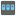• # I have no idea why "For does'nt work

## Question related to missionHouse Password

""" In the for loop below, ncount +=1 ucount += 1 l_count += 1 dosen't work.

I have no idea. Need help.

"""

```def checkio(data: str) -> bool:
n_count = 0
u_count = 0
l_count = 0

check = data
if len(check) > 10:
for char in check:
if '1234567890' in char:
n_count += 1
if 'QWERTYUIOPASDFGHJKLZXCVBNM' in char:
u_count += 1
if 'qwertyuiopasdfghjklzxcvbnm' in char:
l_count += 1

if n_count > 0:
if u_count > 0:
if l_count > 0:
r = True
else:
r = False
else:
r = False
return r
```1